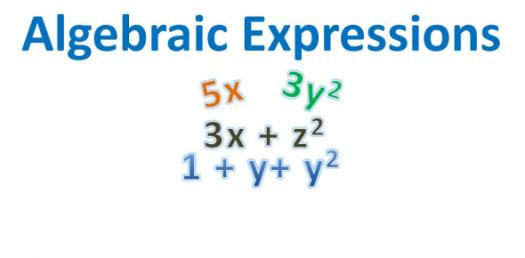# Test On Algebraic Expression! Quiz

6 Questions | Total Attempts: 85SettingsIf you have been having a hard time when it comes to algebra, then this Test on Algebraic Expression is precisely what you may need. Making the right calculations can be a little hard for most of us, but with some practice, one can perfect their skills. Be sure to tackle the exam to perfect your ability to perfect your skills.

• 1.
Divide   -10/40
• A.

-4

• B.

-1/4

• C.

4

• D.

30

• E.

.25

• 2.
Which of the following is irrational?
• A.

Sqrt(25)

• B.

3.454554555...

• C.

3.45455

• D.

1/7

• E.

0

• 3.
Evaluate -(-x) for x = -17
• A.

17

• B.

-16

• C.

16

• D.

-17

• E.

18

• 4.
Evaluate 5s - 3t for s = -14 and t = 13
• A.

25

• B.

31

• C.

-31

• D.

-109

• E.

None of the above

• 5.
Simplify:  x - 3(y - x) =
• A.

-2x -3y

• B.

4x - 3y

• C.

-3y

• D.

Y - 3

• E.

2x - 3y

• 6.
Solve for x:  8 - 5x = 11x - 8
• A.

X = -16/6

• B.

X = 0

• C.

X = -1

• D.

X = 16/6

• E.

None of the above

Related TopicsBack to top